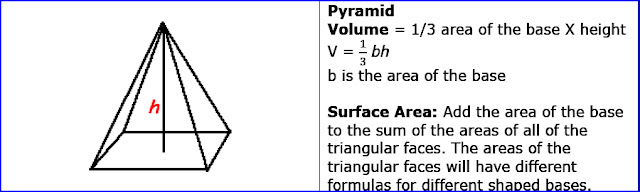# C++ Exercises

## C++ Program to calculate the volume of the Pyramid.

Let see the program to calculate the volume of the Pyramid.

```
Example:

Formula:Pyramid

```
```
/* to calculate the volume of the Pyramid. */
#include <iostream>

using namespace std;
int main()
{
float base,height,volume;

cout<<"Enter base of Pyramid :";
cin>>base;
cout<<"Enter height of Pyramid :";
cin>>height;
volume=(1/3)*base*height;
cout<<"volume of Pyramid = "<<volume<<" units."<<endl;

return 0;
}
```
##### Output:
```
Enter base of Pyramid :1
Enter height of Pyramid :1
volume of Pyramid = 0.33333333 units.

```